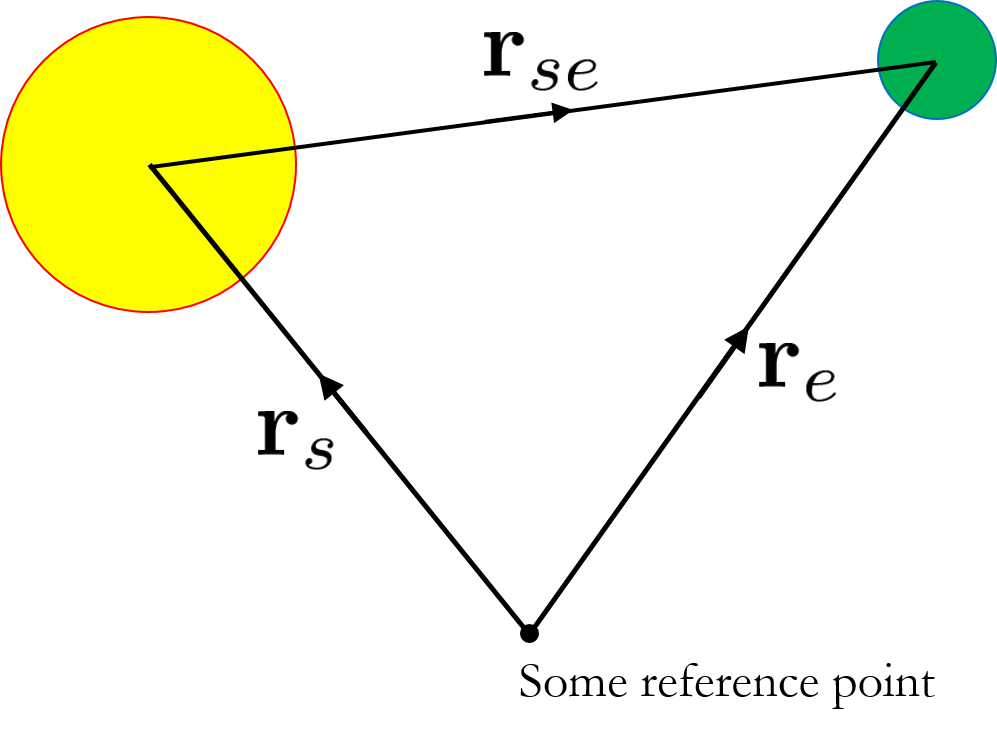Fill out the following (feel free to Google):

 Radius of Sun ($$R$$) $$6.96\times10^{8}$$ m Average distance from Sun to Earth ($$R_{e}$$) $$1.50\times10^{11}$$ m Mass of Sun ($$M_{s}$$) $$1.99\times10^{30}$$ kg Mass of Earth ($$M_{e}$$) $$5.97\times10^{24}$$ kg Gravitational constant ($$G$$) $$6.67\times10^{-11}$$ m3kg-1s-2 Linear momentum of Earth ($$p_{e}$$) $$3\times10^{4}$$ kg ms-1

(We can get $$p_{e}$$ by knowing that the Earth circles around the Sun in about 365 days. How?)Let $${\bf r}_{s}$$ and $${\bf r}_{e}$$ be the position vectors of the Sun and Earth respectively. Express $${\bf r}_{se}$$ in terms of the two postition vectors. ${\bf r}_{se}={\bf r}_{e} - {\bf r}_{s}$

 Newton’s Law of Gravitation: $$\displaystyle {\bf F}=-\frac{GMm}{r^{2}}\hat{{\bf r}}$$ Force that the Sun exerts on the Earth: $$\displaystyle {\bf F}_{se}=-\frac{GM_{s}M_{e}}{r^{2}_{se}}\hat{{\bf r}}_{se}$$ Force that the Earth exerts on the Sun: $$\displaystyle {\bf F}_{es}=\frac{GM_{s}M_{e}}{r^{2}_{se}}\hat{{\bf r}}_{se}$$ Newton’s second law (express in terms of rate of change of momentum) $$\displaystyle {\bf F}=\frac{d{\bf p}}{dt}$$

Applying Euler’s scheme for solving differential equation numerically

\begin{align*} d\textbf{p} &= \textbf{F}dt \\ \textbf{p}_{i + 1} &= \textbf{p}_{i} + d\textbf{p} \\ &= \textbf{p}_{i} + \textbf{F}_{i}dt \end{align*}

Relationship between momentum and position vector is given by:

${\bf p}=m\frac{d{\bf r}}{dt}$

Applying Euler’s scheme for solving differential equation numerically.

\begin{align*} d\textbf{r} &= \frac{\textbf{p}}{m} dt \\ \textbf{r}_{i + 1} &= \textbf{r}_{i} + d\textbf{r} \\ &= \textbf{r}_{i} + \frac{\textbf{p}}{m}dt \end{align*}

We will discuss the above briefly in class and do part 3 together as a group.

To be continued…